## Sunday, September 26, 2010

### setiQuest Kepler-4b redux

The discovery of an extremely interesting FSK modulated signal was reported in my setiQuest Kepler-Exo4 1420 MHz blog entry back on April 24 2010. This finding generated a great deal of excitement and skepticism. Was it a real extraterrestrial transmission from the exoplanet Kepler-4b or was it just local RFI? The SETI Institute decided to follow up and take a second look at the Kepler-04 target in May 15 2010. Two new datasets of "good" observations were released a couple days ago.

The baudline signal analyzer is going to explore this setiQuest Kepler-4b redux data. The base frequency of the two data files are 1418.0 and 1420.0 MHz with an 8.7381 MHz bandwidth. The format is the familiar signed 8-bit quadrature data with a sample rate of 8738133.333 samples/second.

The following command line was used to stream the Kepler-4b data files into baudline:

cat 2010-05-14-kepler04-3* | baudline -session setiquest -stdin -format s8 -channels 2 -quadrature -flipcomplex -samplerate 8738133 -fftsize 65536 -pause -utc 0

First the kepler04-3 dataset will be analyzed and then the kepler04-4 dataset.

Kepler04-3
Date: May-14-2010
Start time: 10:50
Freq: 1418.0 MHz
RA,Dec: 19.041021,50.135753

The kepler04-3*.dat files are the first data set in this redux. A Welch windowed 65536 point FFT for a 266.67 Hz/bin resolution was used to create the image below. Click on image for a larger view.Here is the Average spectrum from a prototype version of baudline that has more sensitivity.Targets:
• -2422400 Hz | 215 sigma | "off the top"
• -1312794 Hz | 6 sigma
• -1274844 Hz | 7 sigma
• -44514 Hz | 4 sigma
• -38376 Hz | 4 sigma
• +2044295 Hz | 22 sigma | "left of Hydrogen"
• +2416146 Hz | 10 sigma | "in Hydrogen"
• +3008373 Hz | 7 sigma | "right of Hydrogen"
There are several signals within the +2044295 Hz "left of Hydrogen" peak so let's decimate by 256 and see what Auto Drift can find: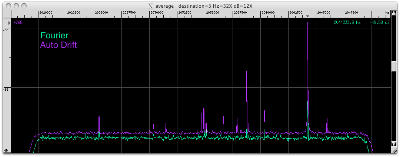Compare the green and purple spectrum curves. Auto drift increases the strength of drifting signals, reduces the variance of the noise floor, and allows the drift rate to be queried with the auto drift rate measurement window.

Drifting Targets:
• +2025472 Hz | 22 sigma | +0.0447 Hz/s
• +2030395 Hz | 6 sigma | +0.0249 Hz/s
• +2031496 Hz | 7 sigma | -0.5453 Hz/s
• +2034730 Hz | 14 sigma | -0.0976 Hz/s | "LSB?"
• +2034911 Hz | 30 sigma | -0.2942 Hz/s | "carrier?"
• +2035122 Hz | 14 sigma | +0.0137 Hz/s | "USB?"
• +2036686 Hz | 12 sigma | -0.1494 Hz/s
• +2037900 Hz | 11 sigma | -0.3151 Hz/s
• +2038257 Hz | 4 sigma | -0.1672 Hz/s
• +2038767 Hz | 45 sigma | -0.0447 Hz/s
• +2038960 Hz | 7 sigma | -0.0991 Hz/s
• +2040367 Hz | 16 sigma | +0.0417 Hz/s
• +2044250 Hz | 78 sigma | -0.1855 Hz/s | "left of Hydrogen"
• +2045996 Hz | 14 sigma | +0.3710 Hz/s
Now let's zoom in, take a look around, and see what we can find.

Hydrogen has Sidebands
Hydrogen is the largest peak in the center (+2526 kHz) of the Average display below: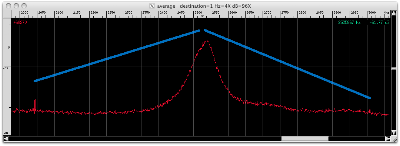Adjusting for Hydrogen's slightly lopsided shape moves the center of mass -14 kHz to the left. When this offset is applied the corrected center of mass sits directly in the middle of the two tones with a delta of ±483 kHz.

-2422400 Hz
Very strong tone. Decimating by 4096.The center tone is measured to be at -2422399.90 Hz. This is a very suspicious number because -2422399.90 / 8738133.33 * 8192 = -2270.99991 which is the whole number -2271 for all practical purposes. I suspect bin is buried somewhere deep in the ATA DSP code, possibly as a tuning parameter.

The purple sidebands are ±73.95 Hz from the center tone. The green sidebands are ±50.00 Hz from the center tone. The two sets of non-harmonically related sidebands suggest that there are two independent distortion/modulation forces (50 & 74 Hz) at work.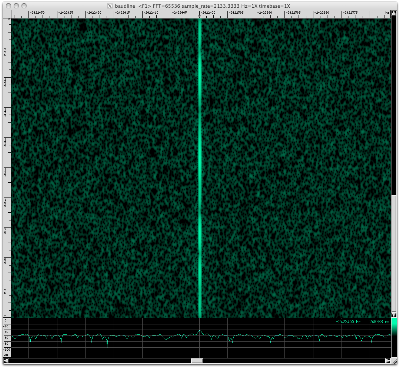A stationary tone with zero drift rate. Four amplitude dropouts of about -14 dB are visible.

While baudline was recording this decimated signal I noticed that the Histogram window underwent some odd and unusual behavior. Here a 3 snapshots:It looks like the DC value of the quadrature channels is changing. To get a better understanding of this dynamic behavior let us look at the problem from a different perspective. Below is the spectrogram of the Histogram transform. The green and purple colors represent the I & Q channels respectively.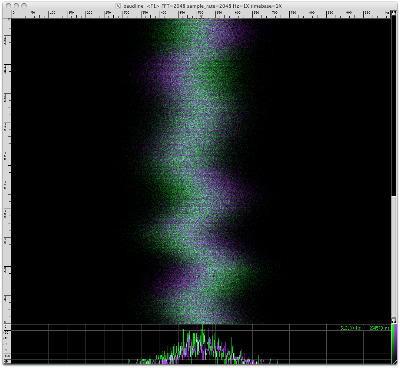One way of interpreting the data is that some sort of strange phasing is at work. Another way of looking at the data is that the quadrature balance is wandering.

-1312794 Hz
Decimating by 4096.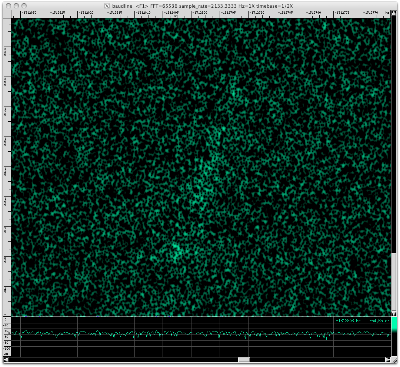Drifting wideband noise with a -8.92 Hz / 112.3 seconds = -0.0794 Hz/sec drift rate.

-1274844 Hz
Decimating by 4096.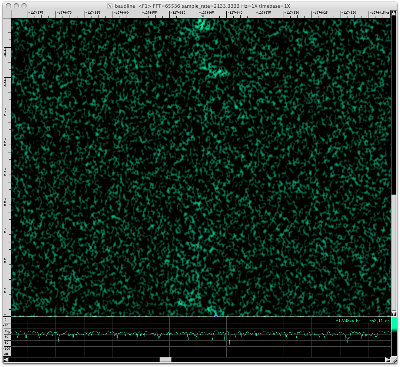Non-drifting wideband noise similar to the previous signal above. The scattered high energy blips are about +10 dB above the noise floor.

-44514 Hz
Decimating by 4096.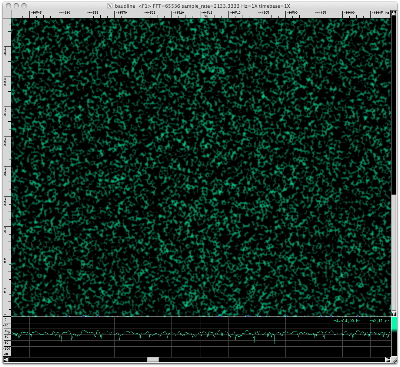More weak non-drifting wideband scatter noise. Four sigma above the noise floor. Not much to see.

-38376 Hz
Decimating by 4096.Another 4 sigma signal. Weak non-drifting wideband scatter noise. Not much to see.

+2025472 Hz
First signal from the Auto Drift target set. Decimating by 4096.A drifting random walk with a +10.81 Hz / 255.7 seconds = +0.0423 Hz/sec drift rate. Using baudline's periodicity bars shows a repetitive 25 Hz frequency bounce. Further inspection shows a roughly 2.5 Hz pulsing.

+2030395 Hz
Decimating by 4096.A weak drifting random walk with a +5.92 Hz / 255.7 seconds = +0.0232 Hz/sec drift rate.

+2031500 Hz

Decimating by 4096. Note that the frequency axis has been changed to Hz=4X so the entire signal fits on the screen.A drifting random walk with a -125.26 Hz / 255.7 seconds = -0.4899 Hz/sec drift rate. The periodic bars found a rough periodicity of 28.4 seconds.

+2034731 Hz
This signal looked like a lower sideband (LSB) to a nearby carrier. Decimating by 4096.A drifting random walk with a -24.41 Hz / 255.7 seconds = -0.0955 Hz/sec drift rate. Blip pulsing with a 7.48 second periodicity.

+2034913 Hz
This signal looked like a carrier that had upper and lower sidebands. Decimating by 4096. Note that the frequency axis has been changed to Hz=2X so the entire signal fits on the screen.A drifting random walk with a -70.83 Hz / 255.7 seconds = +0.2770 Hz/sec drift rate.

+2035122 Hz
This signal looked like an upper sideband (USB) to a nearby carrier. Decimating by 4096.A drifting random walk with a +2.21 Hz / 255.7 seconds = +0.00864 Hz/sec drift rate. Blip pulsing with a 5.7 second periodicity. Here is a zoomed out spectrogram that shows the relationship of the previously mentioned LSB-carrier-USB signals:The "LSB", "carrier", and "USB" signals all looked to be related in the Average spectrum with ±200 Hz spacings but they have different drift rates and aren't sidebands at all. This shows the importance of different perspectives. What looks like something in one visualization might have different characteristics in another viewpoint.

+2036687 Hz
Decimating by 4096.A drifting random walk with a -37.89 Hz / 255.7 seconds = -0.1482 Hz/sec drift rate.

+2037910 Hz
Decimating by 4096. Note that the frequency axis has been changed to Hz=2X so the entire signal fits on the screen.A drifting random walk with a -69.40 Hz / 255.7 seconds = -0.2714 Hz/sec drift rate. A weak 27 second periodicity similar to what was seen above at +2031500 Hz.

+2038261 Hz
Decimating by 4096. Note that frequency axis is set to Hz=2X.Two trajectories with a split point at 100 seconds mark near the middle. The upper section has a -25.65 Hz / 155.7 seconds = -0.1647 Hz/sec drift rate. The lower section has a -13.15 Hz / 100 seconds = -0.1315 Hz/sec drift rate.

+2038767 Hz
Decimating by 4096.A drifting random walk with a -12.30 Hz / 255.7 seconds = -0.04810 Hz/sec drift rate.

+2038960 Hz
Decimating by 4096.A drifting and oscillating random walk with a -43.95 Hz / 255.7 seconds = -0.1719 Hz/sec drift rate. The drift motion looks like a full cycle of a sinusoid.

+2040365 Hz
Decimating by 4096.A drifting random walk with a +10.34 Hz / 255.7 seconds = +0.04044 Hz/sec drift rate.

+2044295 Hz
Hydrogen's left sideband. The distance from Hydrogen's center of mass is -483 kHz. Decimating by 4096.A drifting random walk with a -43.10 Hz / 255.7 seconds = -0.1686 Hz/sec drift rate.

What at first looked like a drifting random walk in fact has periodic structure. Pay attention to the short vertical discontinuous lines that all appear to have a duration of ~4.5 seconds. It looks binary and has FSK characteristics but I have never seen a modulation implementation like this. Using baudline's periodicity bars shows how these vertical bars line up.

The clocking appears to be very stable. The periodicity bars synced up all 21 vertical discontinuous lines for a 4.503 second duration for a 0.2221 baud rate. Coding the vertical discontinuous bars as the "1" symbol and slanting wiggling drift as the "0" symbol results in this demodulated string of 54 symbols:

10101011000001010100000001101100000110(1000010101101010

The "(" symbol represents an error because the slope briefly reversed direction and became positive, hence its shape looks like a parenthesis. Statistically the "(" symbol most likely is a "1" symbol.

Using a context-free grammar to break down the demodulated string yields:

3(10) 11 5(0) 3(10) 6(0) 11011 5(0) 110(1 4(0) 2(10) 110 2(10)

Runs of "10" and "0" appear common, while "11" and/or " "11011" are less frequent and may be separators or sync codes.

As previously mentioned this modulation scheme is unusual. One way of generating this signal would be to have a drifting tone that periodically stops drifting for 4.5 seconds and then restarts ("drift-n-drop"). [Update: Zooming in shows that the vertical drops are not exactly stationary.] To look at this from a different frame of reference let us correct the steep drift rate by rotating the image by about 40° in a graphics editor.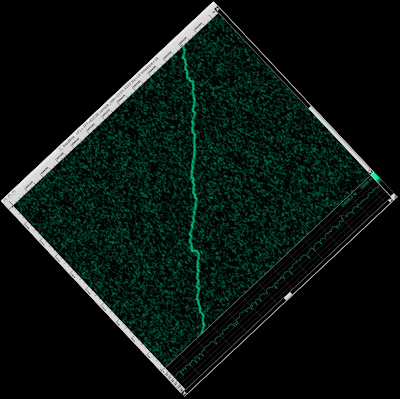In this point of view the signal shape takes on a wandering zigzag shape that has a very sawtooth-like appearance. In this rotated view the binary transitions are more pronounced. Now this was a cute graphical demonstration of what we are going to do next in the audio DSP domain.

Now let us properly drift correct this modulated signal using baudline's many DSP manipulation features as building blocks. Multiple instances of baudline need to be connected together to form a multi-pass processing chain. This can be done by using the -stdin / -stdout command line options, JACK, SoundFlower, or the digital loop mode of an Edirol UA-25 USB device.

Here are the basic steps:
1. Set the Tone Generator to output a sine wave that is FM modulated by the ramp up function.
2. Set the mix (x*y) operation in the Channel Mapping window.
3. In one baudline instance play the decimated drifting signal at half speed with the Play Deck, record this stream in a second instance of baudline. You now have the intermediary drift corrected mix spectrogram image shown below.
4. Begin analysis by playing this drift corrected mixed signal to another instance of baudline. Use the Play Deck's LPF and HPF controls to create a bandpass filter, or use baudline's decimating down mixer to filter out the carrier and lower sideband.
Intermediary image of the drift corrected "mix" spectrogram is a visual description of the DSP processing involved:

The purple ramp up FM modulated sine wave is mixed (x*y) with the original signal to create a folded lower sideband and a straightened upper sideband. The upper sideband is extracted by using LPF/HPF filters or the decimating down mixer as a bandpass filter. Note that the Play Deck's speed and shift sliders can be used to adjust the spectral resolution and position. The Play Deck tools can also be used to listen to this signal.

Here is the extracted drift-corrected signal:

We now have a drift corrected version of this modulated signal. Note that the correct sample rate is actually 531.956 samples/second. I see some signal features that I missed during the first look. Let's zoom in and explore.

Decimate by another factor of 32 for a 0.00818 Hz/bin resolution.

This looks like a hybrid modulation of pulses on a zigzag with groupings of FSK. It is interesting that the frequency before each zig and zag is approximately the same after. It is as if the creating machinery after each zigzag wants to return to a moving centroid.

The periodicity bars will make this concept clearer.

A fairly stable clocking with a periodicity of 1 / 49.239 seconds = 0.020309 symbol rate. The pattern is 0+0-0+0. The 3 states suggest that the zigzag modulation could be some form of trinary (base-3). The lack of positive to negative transitions {+-, -+} suggest a differential coding scheme but unfortunately 6 1/2 symbols isn't enough information to infer details with any level of confidence.

Now let's use the periodicity bars again to measure the clocking rate of the FSK symbols. A smaller 1024 point FFT size was used along with the blip Fourier transform in magnitude space of a bit more resolution.

The straighter pulse sections ( for example the 175 second position) clock perfectly with a periodicity of 1 / 4.9112 seconds = 0.20362 symbol rate. The faster FSK sections are off in an unusual symbol-and-a-half way. I mean every other symbol is sliced perfectly in the middle. Let's try again and focus on the faster FSK sections.

All of the faster FSK sections clock perfectly with a periodicity of 1 / 3.2884 seconds = 0.30410 baud rate but the slower pulsed sections are now sliced incorrectly. So we are in the unpleasant situation where both symbol rates slice different sections of the modulated signal perfectly but fail when applied to the signal as a whole. One way to explain this is that the signal is switching between two different symbol rates which is a very unusual thing to do. It would make demodulation much more difficult but it might be beneficial (speculatively) by adding a second embedded clock which could provide some added ISM distortion immunity. Another way of explaining the two symbol rates is that both are wrong and the correct answer is a faster rate that is a least common multiple (LCM) of the two. Let's zoom in and try again with something in the 0.6 symbols/second range.

Eureka! We have perfect clocking with a periodicity of 1 / 1.6457 seconds = 0.60764 symbol rate. The result does look strange with the slower pulses being triple sampled and the faster FSK sections being double sampled. It seems like a waste and I doubt it is a result of the binary data sequence. It could be an error redundancy scheme but I'm not going to speculate. What is important is that this faster symbol rate solves the data periodicity clocking perfectly.

The delta between mark and space frequencies is about 0.3 Hz. Using a bandwidth of twice the mark/space delta (Nyquist) the spectral efficiency is 0.30410 baud / 0.6 Hz = 0.507 (bit/s)/Hz. Using the same bandwidth rule, the spectral efficiency of the FSK signal in the original Kepler-4 analysis is 0.5061 baud / 2.4 Hz = 0.211 (bit/s)/Hz which is a little less than half of what is seen here. So roughly half the baud rate, a fourth the bandwidth, for about double the spectral efficiency. So it scaled in a squared sort of way.

A good question is what are the FSK bits doing in the zigzag sections? Maybe isolated FSK sections aren't the correct way to interpret the data? It could be a trinary scheme were the motions are either a {-, 0, +} ∆f adjustment instead of mark/space hopping? This would correspond with the first zigzag trinary modulation we saw with the slow 0.02 symbol rate.

Now we are going to investigate any possible phase modulations by using the blip Fourier transform set to phase space. The blip transform incorporates a blind phase locking algorithm that is ideal for this sort of work. The Play Deck's LPF/HPF controls were used to create a narrow bandpass filter. Decimation was set to 2 so that the down mixer could be used to adjust the frequency center. Note the 265 samples/second rate is 16X higher than the previous modulation analyses. Ignore the reddish orange colors and focus on the vertical blue band near the arrow at 61 Hz. The blue color fluctuations represent a change of phase.

What we see here are 4 distinct phases (0°, 90°, 180°, 270°) that suggests a QPSK modulation scheme. The blueish "cos full" color palette was used because it helped reduce the visual phase ambiguity.

Next let's use baudline's periodicity bars to explore the modulation clocking.

Perfect symbol placement with a very stable clocking periodicity of 1 / 0.10678 seconds = 9.3649 symbol rate. Here is a demodulation of the phase symbols with the codes dark to light ={ 0,1, 2, 3 }.

202222120301202112113021012213020203

36 symbols with no consecutive runs of 0's or 3's. There is not enough data to make much of this and as always my thresholds could of been off so demodulation errors are a definite possibility. We see 4 phases but is this really QPSK? Let us take a look with the Waveform window. Here is a random segment from the data file:

The two interesting features are what looks to be wave packets and the two quantized amplitude levels. The two energy levels suggest that this modulation is Quadrature Amplitude Modulation (QAM). So what we thought was QPSK becomes 8-QAM which nobody uses since 8-PSK is more efficient. To get a slightly different perspective let us look at the same data segment we analyzed above for the phase spectrogram but this time we'll look with the blip Fourier transform in magnitude space.

This is left as an exercise for the reader. Aligning the periodicity bars up at the 9.365 symbol/sec rate again shows perfect modulation clocking but the symbols now take on 3 amplitude levels (bright red, medium red, and blue). So what looks like empty blue space between the red packets are in fact symbols. So what we previously thought was 8-QAM now has 4 distinct phase and 3 amplitudes. Here is a demodulation of the amplitude symbols with the codes high to low = { a, b, c }.

aaaaaabbbbacbccbbbbbbaabbabbbbbbcbcc

What stands out are the three independent runs of six consecutive symbols (a's and b's) and the two cbcc sequences. Placing the demodulated phases and amplitudes symbols next to each other gives us a QAM representation.

202222120301202112113021012213020203
aaaaaabbbbacbccbbbbbbaabbabbbbbbcbcc

The QAM phase/amplitude pairs should be read as columns { 2a, 0a, 2a, 2a, ...}. Assuming all the possible phase/amplitude combinations are populated we now have 12-QAM which not a common modulation scheme. This strange modulation could be due to analysis misinterpretation or it could be that the scheme is QAM-like but something slightly different. The only way to know for sure is to look at it in a constellation window. [Great, now I need to create another baudline tool!]

As a sanity check of the blip phase locking algorithm here is the Waveform window with the periodicity bars set to a 9.365 symbol rate. The phase of the sinusoid does jump around in accordance to what the blip Fourier transform predicts. Cool.

The 3 different modulation types (zigzag trinary, FSK pulsing, and QAM) layered on top of each other is extremely unusual. What is the significance of a modulation in a modulation in a modulation? That's modulation^3. I've never seen anything like this before. How could this be created? For what purpose?

Here is a summary of the 3 modulation types and their respective symbol rates:
• zigzag trinary | Z = 0.020309 symbol rate (error ±0.00004)
• FSK pulsing | F = 0.60764 symbol rate (error ±0.001)
• 12-QAM | Q = 9.3649 symbol rate (error ±0.01)
Here are ratios comparing the symbol rates:
• F / Z = 0.60764 / 0.020309 = 29.920
• Q / F = 9.3649 / 0.60764 = 15.412
• Q / Z = 9.3649 / 0.020309 = 461.12
The ratios round to the integers 30, 15, and 461 which factor to 2 * 3 * 5, 3 * 5, and prime respectively. While baudline's periodicity bars can make hyper-accurate measurements the margin of error is large enough that Q/Z could be 460. So any extra emphasis placed on a prime ratio value is likely unjustified. What is significant is how the symbol rate scales as the modulation complexity increases, first by 30 and then by about 15.

Next let us look at the Autocorrelation transform which is a measure of self-similarity. A 4096 point FFT was used.

Autocorrelation with a 8192 point FFT and a slightly narrower Kaiser window.

The 8192 point Autocorrelation version is sharper while the 4096 point version looks rougher and more grainy which most likely is due to the decreased FFT gain. This tells us that the periodic signals at work here are fairly local in time.

There is a lot of structure in both Autocorrelation spectrograms with the placement and spacing of the holes being the most intriguing feature. The holes I believe are a result of the hybrid FSK-pulse-like modulation. There are a couple groups of what could be 10101010... symbols near the top and middle. Near the bottom are two slightly offset groups of 100100100... symbols. Both repetitive patterns could be training or sync sequences. Many random holes are scattered about in the middle and they could represent the data payload. It is extremely difficult to extract any meaning from 50 bits (symbols?) so this is all speculation.

An important observation is how similar the holes look like those seen in the original Kepler-4 Autocorrelation analysis. [More comparison and analysis is needed to determine if this similarity can be construed as matching modulation markers. I'm delving into forensic signal analysis here. The question I am asking is were both Kepler-4 modulated signals created by the same process or machine? (as in the same modem or the same RFI mechanism)]

+2045991 Hz
Decimating by 4096. Note that frequency axis is set to Hz=2X so that the full drift is visible.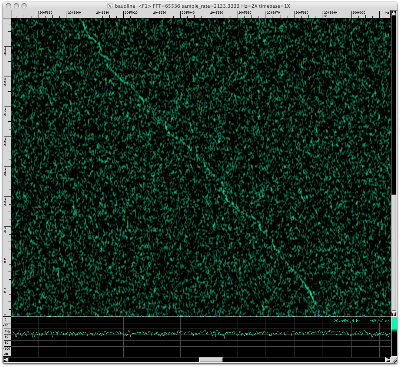A drifting random walk with a +85.29 Hz / 255.7 seconds = +0.3336 Hz/sec drift rate. The drift looks like it has some oscillations with 54 second period.

+2416146 Hz
Signal in Hydrogen. Decimating by 4096.Non-drifting wideband noise. The scattered high energy blips are about +10 dB above the noise floor.

+3008373 Hz
Hydrogen's right sideband. The distance from Hydrogen's center of mass is +483 kHz. Decimating by 4096.Drifting wideband noise with a +62.76 Hz / 255.7 seconds = +0.2454 Hz/sec drift rate. The banded noise has upper and lower segments with a scattering of several high energy blips (narrow in time and frequency).

Kepler04-4
Date: May-14-2010
Start time: 11:04
Freq: 1420.0 MHz
RA,Dec: 19.041021,50.135753

The kepler04-4*.dat files are the second data set in this redux and they have a different base frequency and a slightly later start time. A Welch windowed 65536 point FFT for a 266.67 Hz/bin resolution was used to create the image below.Targets:
• +44234 Hz "left of Hydrogen"
Many of the other signals are in the Kepler04-4 data set but only the +44234 Hz modulated candidate signal will be verified.

+44234 Hz
This signal is -486 kHz left of Hydrogen's center of mass. Decimating by 4096.A drifting random walk with a -39.26 Hz / 322.4 seconds = -0.1218 Hz/sec drift rate. Baudline's periodicity bars again show a ~4.5 second periodicity.Here is the drift corrected spectrogram:

A very similar trinary/differential modulation shape. The periodicity bars measured a 51.353 second symbol rate which is close to the previously measured 49.239 periodicity. How does this compare to the modulated signal at +2044295 Hz seen in the first Kepler04-3 dataset? The drift rate, drifted starting frequency, top-layer modulation scheme and symbol rate all match so the signal in this dataset is a continuation of the first.

TBD
This blog post is a work-in-progress. I might even add a movie for the zigzag modulated signal at +2044295 Hz. Check back soon.

Conclusion
A total of 21 signals were found in the Kelper04-3 data set. There was some wideband scatter noise and many drifting random walks. A few of the drifting random walks displayed interesting periodicities. The drifting +2044295 Hz signal exhibited unique modulation structure and was investigated in great detail.

Let us compare that interesting FSK signal discovered in the original Kepler-04 analysis done back in April to the unusual zigzag signal found today at +2044295 Hz. The 2010-01-22-kepler-exo4 FSK signal was approximately -480 kHz left of Hydrogen, had a +0.0132 Hz/sec drift rate, and a 0.5064 baud rate. The 2010-05-14-kepler04-(3)(4) zigzag "drift-n-drop" signal is -483 kHz from Hydrogen's center of mass, has a -0.1686 Hz/sec drift rate, and 0.2221 baud rate. They have very different signal characteristics but they several important things in common:
• same RA/Dec (celestial position)
• distance left of Hydrogen (-480 kHz)
• drifting in frequency
• matching FSK modulation markers
• low modulated baud rate
• similar Autocorrelation shapes
All of the above seems like too much of a coincidence to be random happenchance. Does this qualify as verification of the January Kepler-04 FSK signal? I think it does. Robackrman of the SETI Institute doesn't agree and thinks it is an "imprecise and wandering carrier." Robackrman then uses a Pulsar B0809+74 data set recorded later that day as an OFF signal and shows the presence of the drifting zigzag FSK signal. This suggests that the FSK signal is RFI. I am waiting for the SETI Institute to post this data set so I can confirm these findings.

On 9-24-2010 the SETI Institute took a third look at Kepler-4b and this data has now been posted. I will be taking a look at it soon.

A quick scan of my old setiQuest reports shows that the pulsar PSR B0329+54 analysis found an unusually modulated signal that was located -482 kHz left of Hydrogen. Hmmmm.